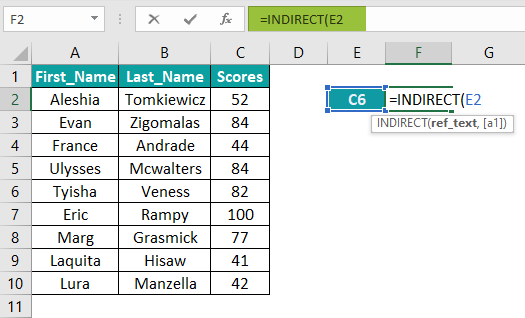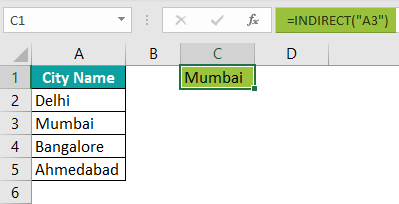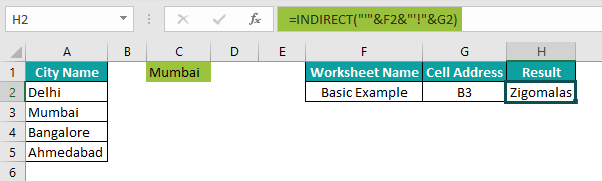# INDIRECT Excel FunctionArticle byExcelMojo Team## What Is INDIRECT Function In Excel?

INDIRECT function in Excel helps users reference cells, ranges or arrays, and even worksheets and workbooks indirectly. The function takes the cell reference as the text string and returns the value in the given cell reference. Thus, it returns the cell reference specified by the text string.

The INDIRECT function allows users to update a reference within a formula without changing the formula. For instance, we have a fruits list in the Excel sheet below:

We will try to retrieve the value from cell A4 by entering the below formula in cell C2. The INDIRECT function in Excel takes reference for cell A4 in the form of string “A4” and returns the value present in cell A4.

=INDIRECT(“A4”)

###### Key Takeaways
• The INDIRECT function in Excel retrieves the cell reference from the given text string.  As a result, it returns a range reference.
• One can update a reference within a formula without changing it.
• The INDIRECT function uses both R1C1 and A1 style references and requires TRUE or FALSE as the last argument.
• It can be used to make a dependent drop-down list, but it cannot be used to assess logical tests or conditions.

### Syntax – INDIRECT Excel Formula

Below is the syntax of using INDIRECT function in Excel.

The INDIRECT function syntax has two parameters where one is mandatory, and the remaining one is optional.

• ref_text: This is a cell reference, reference cell, or a named range as a text string. The reference should be valid; otherwise, the function will return a #REF error. It is a mandatory argument.
• [a1]: This is a logical value specifying the reference type for the first argument ref_text. It is an optional argument. We can give either TRUE or FALSE.
• TRUE – This will interpret ref_text as an A1-style reference.
• FALSE – This will interpret ref_text as an R1C1-style reference.

If nothing is specified, it will take TRUE as the default argument.

### How To Use INDIRECT Excel Function?

Let us look at a basic example of using the INDIRECT function in Excel.

Below is the data set of students and their scores:

First, we will try to find the value present in cell C6.

We will enter the C6 cell reference value in cell E2.

In the above example, we have entered the cell reference in double-quotes directly to the INDIRECT formula, but here we have a cell reference string in cell E2.

Follow the below steps to use the INDIRECT excel function.

1. Enter the INDIRECT function in cell F2.2. Choose cell reference value in cell E2.3. Since we are using an A1 style reference, there is no need to mention TRUE. Ignore the last argument. However, by default, it takes TRUE.

4. Close the bracket and hit the ‘Enter’ key to get the result.The INDIRECT function took the cell reference to cell C6 as a string indirectly from cell E2 and returned the value from cell C6.

When we enter cell text string value, there is no need to mention double quotes because cell E2 stored the C6 value in string format by default.

### Examples

Let us look at some advanced INDIRECT function in excel examples to understand how it works:

#### Example #1: Indirect Cell Reference Using R1C1 Style

We have seen the A1 style reference in the above example, and here, we will see the R1C1 style reference.

Consider the same above data here as well.

Assume that we need to retrieve the value from cell C9 using the R1C1 style reference. In R1C1 style, R stands for ROW, and C stands for COLUMN.

Step 1: Since we are looking for the cell C9 value, the row will be 9, and the column will be 3. Hence, the R1C1 style will be R9C3.

Enter this reference in any of cell E1.

Step 2: Enter the INDIRECT function in cell F1.

Step 3: Choose cell E1 for the argument ref_text.

Step 4: Since we use the R1C1 style reference, we need to mention FALSE for the argument [a1].

Close the bracket and hit the ‘Enter’ key to get the result in cell F1.

The result in cell F1 is 41, which is the value we have in cell C9.

#### Example #2: INDIRECT Function With Multiple Ranges

As of now, we have seen a single cell reference with the INDIRECT function. However, the function can be used for multiple ranges as well.

Below are the monthly sales of products in a department store.

Assume we need to get the total sales for the product “Banana” using the INDIRECT function.

Enter the INDIRECT function in cell F2.

Enter the cell reference for Banana as “C2:C6” in double quotes.

We can ignore the last argument since we provide an A1-style reference.

Close the bracket and hit the ‘Enter’ key to get the result.

We have got all the values in a range of cells C2:C6. We need to wrap the INDIRECT function with the SUM function to get the sum of these numbers.

Enter the SUM function before the INDIRECT function.

The resulting value of 11,422 is the total of the product Banana.

However, this is not the dynamic way of getting the sum. If we want to get the sum for another product, we must manually change the reference. We will see a dynamic way to get the result in the below example.

#### Example #3: Dynamic Results with Named Ranges

In excel, we can create named ranges. For instance, we can enter “Product_Sales” for the above table.

Then, select the entire table range.

In the Name box, enter the name “Product_Sales”.

Now, we can use this named range in the INDIRECT function. Enter the ‘named range’ in cell F1.

Enter the INDIRECT function in cell F2 and give the F1 cell reference.

Close the bracket and hit the ‘Enter’ key to get the result.

The INDIRECT function has returned the whole table because the named range “Product_Sales” has cells reference A1:D6.

Similarly, we can do dynamic calculations for each product. Follow the below steps:

Step 1: Create a named range for each product, as shown below.

We have three named ranges Carrot, Banana, and Potato.

Enter any of this named range in cell F1.

Enter the INDIRECT function in cell F2 and choose the F1 cell.

Close the bracket and hit the ‘Enter’ key.

We have got the all the month’s sales value for the product “Carrot”.

Now, wrap the INDIRECT function with the SUM function.

We have got the total sales for the product “Carrot”.

This formula is dynamic, we can change the product name in cell F1, and accordingly, the formula returns the total sales for the entered product in cell F1.

#### Example #4: Create Dependent Drop-Down List

The INDIRECT function in Excel can be used to create a drop-down list. The dependent drop-down list is nothing but a list of one cell depending on the value entered in the other cell.

For instance, we have a list of cricket players from each country in an excel spreadsheet.

We will try to create two drop-down lists – one for the country and another for players. Players’ drop-down list depends on the selection in the country drop-down list. For example, if we select “India” in the country drop-down, players’ drop-down should show only player names from India.

Step 1: Give a named range for each country like the below.

Give the name “India” for the range “A2:A6”.

Give the name “Australia” for the range “B2:B6”.

Give the name “South_Africa” for the range “C2:C6”.

Step 2: Select cell E2. Go to the DATA tab and choose ‘Data Validation.’

Step 3: This will open the ‘Data Validation’ window like the below and choose “List” from the Allow drop-down.

Step 4: In the Source box, enter all the country names created in step 1.

Step 5: Click on “Ok,” and a drop-down list is ready for the country in cell E2.

Similarly, now we need to create a drop-down list for players. But this drop-down should depend on the country drop-down created in cell E2.

Step 1: Select cell F2. Go to the DATA tab and choose ‘Data Validation.’

Step 2: This will open the ‘Data Validation’ window and choose “List.”

Step 3: In the Source section, enter the INDIRECT function.

Step 4: We have created a country drop-down list with named ranges names in cell E2, so enter the E2 cell reference for the INDIRECT function.

Step 5: Close the bracket of the INDIRECT function and click on the “Ok” button of the data validation tab.

Step 6: Select any of the countries in the E2 cell drop down, and the F2 cell drop down should show the player’s list for the selected country.

We have selected “India” in cell E2, and in the cell F2 drop-down, we can see only the India players list.

Similarly, we can change the country name in the E2 cell to show the corresponding country players list in the F2 cell.

Like this, by using the INDIRECT function, we can create a dependent drop-down list.

### Important Things To Note

• The INDIRECT function in Excel is volatile and used only when necessary.
• By default, it takes A1 style reference. But if you are using the R1C1 style reference, you have to mention the FALSE in the [a1] parameter.
• Named Ranges should not contain any space or special character except underscore (_).

What is INDIRECT function in excel?

The INDIRECT function allows users to indirectly reference cells, ranges, arrays, and even worksheets and workbooks. The function in excel takes the cell reference in string text format and returns the corresponding value from the given string text cell.

For instance, if we enter the reference of cell A3 in double-quotes, the INDIRECT function will return the city name in cell A3.As we can see above, we have the result as Mumbai because, in cell A3, we have this value.

How to use INDIRECT function in excel data validation?

Using the INDIRECT function, we can create a dependent drop-down list. Please refer to Example #4.

How to use INDIRECT function in excel to reference another worksheet?

The INDIRECT function is used to get the reference from another worksheet. For instance, look at the below example.In cell F2, we have the worksheet name, and in cell G2, we have the cell address of this worksheet. Therefore, to get the value from the B3 cell of the worksheet “Basic Example,” in the INDIRECT function, we need to surround the worksheet name with the single quote “’” and end the quote along with the exclamation (!) symbol and then give the cell reference.

This will return the value from cell B3 of the basic worksheet example.

Why use INDIRECT function in excel?

Whenever we need to create dynamic dependent drop-down lists and retrieve values from indirect cells, the INDIRECT function is very helpful.